• 1、多元线性回归 n 个预测器变量，那么模型可以用以下方程表示： 波士顿房价数据集。该数据集包含 506 座房子的 13 个特征，均值为 $1000。你将用一个模型拟合这 13 个特征，以预测房价 from sklearn.linear_model ... 1、多元线性回归 n 个预测器变量，那么模型可以用以下方程表示：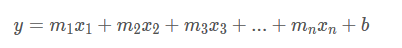波士顿房价数据集。该数据集包含 506 座房子的 13 个特征，均值为$1000。你将用一个模型拟合这 13 个特征，以预测房价
from sklearn.linear_model import LinearRegression

# Load the data from the boston house-prices dataset
x = boston_data['data']
展开全文机器学习
• sklearn实现多元线性回归多项式回归，个人学习笔记，简单易懂，实现多项式回归sklearn
• 本文介绍回归模型的原理知识，包括线性回归多项式回归和逻辑回归，并详细介绍Python Sklearn机器学习库的LinearRegression和LogisticRegression算法及回归分析实例。进入基础文章，希望对您有所帮助。
欢迎大家来到“Python从零到壹”，在这里我将分享约200篇Python系列文章，带大家一起去学习和玩耍，看看Python这个有趣的世界。所有文章都将结合案例、代码和作者的经验讲解，真心想把自己近十年的编程经验分享给大家，希望对您有所帮助，文章中不足之处也请海涵。Python系列整体框架包括基础语法10篇、网络爬虫30篇、可视化分析10篇、机器学习20篇、大数据分析20篇、图像识别30篇、人工智能40篇、Python安全20篇、其他技巧10篇。您的关注、点赞和转发就是对秀璋最大的支持，知识无价人有情，希望我们都能在人生路上开心快乐、共同成长。
前一篇文章讲述了数据分析部分，主要普及网络数据分析的基本概念，讲述数据分析流程和相关技术，同时详细讲解Python提供的若干第三方数据分析库，包括Numpy、Pandas、Matplotlib、Sklearn等。本文介绍回归模型的原理知识，包括线性回归、多项式回归和逻辑回归，并详细介绍Python Sklearn机器学习库的LinearRegression和LogisticRegression算法及回归分析实例。进入基础文章，希望对您有所帮助。

文章目录
一.回归1.什么是回归2.线性回归
二.线性回归分析1.LinearRegression2.线性回归预测糖尿病
三.多项式回归分析1.基础概念2.PolynomialFeatures3.多项式回归预测成本和利润
四.逻辑回归1.基础原理2.LogisticRegression3.鸢尾花数据集回归分析实例
五.本章小结

下载地址：
https://github.com/eastmountyxz/Python-zero2one
前文赏析：
第一部分 基础语法
[Python从零到壹] 一.为什么我们要学Python及基础语法详解[Python从零到壹] 二.语法基础之条件语句、循环语句和函数[Python从零到壹] 三.语法基础之文件操作、CSV文件读写及面向对象
第二部分 网络爬虫
[Python从零到壹] 四.网络爬虫之入门基础及正则表达式抓取博客案例[Python从零到壹] 五.网络爬虫之BeautifulSoup基础语法万字详解[Python从零到壹] 六.网络爬虫之BeautifulSoup爬取豆瓣TOP250电影详解[Python从零到壹] 七.网络爬虫之Requests爬取豆瓣电影TOP250及CSV存储[Python从零到壹] 八.数据库之MySQL基础知识及操作万字详解[Python从零到壹] 九.网络爬虫之Selenium基础技术万字详解（定位元素、常用方法、键盘鼠标操作）[Python从零到壹] 十.网络爬虫之Selenium爬取在线百科知识万字详解（NLP语料构造必备技能）
第三部分 数据分析和机器学习
[Python从零到壹] 十一.数据分析之Numpy、Pandas、Matplotlib和Sklearn入门知识万字详解(1)[Python从零到壹] 十二.机器学习之回归分析万字总结全网首发（线性回归、多项式回归、逻辑回归）

作者新开的“娜璋AI安全之家”将专注于Python和安全技术，主要分享Web渗透、系统安全、人工智能、大数据分析、图像识别、恶意代码检测、CVE复现、威胁情报分析等文章。虽然作者是一名技术小白，但会保证每一篇文章都会很用心地撰写，希望这些基础性文章对你有所帮助，在Python和安全路上与大家一起进步。

监督学习（Supervised Learning）包括分类算法(Classification)和回归算法（Regression）两种，它们是根据类别标签分布的类型来定义的。回归算法用于连续型的数据预测，分类算法用于离散型的分布预测。回归算法作为统计学中最重要的工具之一，它通过建立一个回归方程用来预测目标值，并求解这个回归方程的回归系数。
一.回归
1.什么是回归
回归（Regression）最早是英国生物统计学家高尔顿和他的学生皮尔逊在研究父母和子女的身高遗传特性时提出的。1855年，他们在《遗传的身高向平均数方向的回归》中这样描述“子女的身高趋向于高于父母的身高的平均值，但一般不会超过父母的身高”，首次提出来回归的概念。现在的回归分析已经和这种趋势效应没有任何瓜葛了，它只是指源于高尔顿工作，用一个或多个自变量来预测因变量的数学方法。图1是一个简单的回归模型，X坐标是质量，Y坐标是用户满意度，从图中可知，产品的质量越高其用户评价越好，这可以拟合一条直线来预测新产品的用户满意度。
在回归模型中，我们需要预测的变量叫做因变量，比如产品质量；选取用来解释因变量变化的变量叫做自变量，比如用户满意度。回归的目的就是建立一个回归方程来预测目标值，整个回归的求解过程就是求这个回归方程的回归系数。
简言之，回归最简单的定义就是：
给出一个点集，构造一个函数来拟合这个点集，并且尽可能的让该点集与拟合函数间的误差最小，如果这个函数曲线是一条直线，那就被称为线性回归，如果曲线是一条三次曲线，就被称为三次多项回归。

2.线性回归
首先，作者引用类似于斯坦福大学机器学习公开课线性回归的例子，给大家讲解线性回归的基础知识和应用，方便大家的理解。同时，作者强烈推荐大家学习原版Andrew Ng教授的斯坦福机器学习公开课，会让您非常受益。假设存在表1的数据集，它是某企业的成本和利润数据集。数据集中2002年到2016年的数据集称为训练集，整个训练集共15个样本数据。重点是成本和利润两个变量，成本是输入变量或一个特征，利润是输出变量或目标变量，整个回归模型如图2所示。现建立模型，x表示企业成本，y表示企业利润，h（Hypothesis）表示将输入变量映射到输出变量y的函数，对应一个因变量的线性回归（单变量线性回归）公式如下：那么，现在要解决的问题是如何求解的两个参数和。我们的构想是选取的参数和使得函数尽可能接近y值，这里提出了求训练集(x,y)的平方误差函数（Squared Error Function）或最小二乘法。
在回归方程里，最小化误差平方和方法是求特征对应回归系数的最佳方法。误差是指预测y值和真实y值之间的差值，使用误差的简单累加将使得正差值和负差值相互抵消，所采用的平方误差（最小二乘法）如下：在数学上，求解过程就转化为求一组值使上式取到最小值，最常见的求解方法是梯度下降法（Gradient Descent）。根据平方误差，定义该线性回归模型的损耗函数（Cost Function）为，公式如下：选择适当的参数让其最小化min，即可实现拟合求解过程。通过上面的这个示例，我们就可以对线性回归模型进行如下定义：根据样本x和y的坐标，去预估函数h，寻求变量之间近似的函数关系。公式如下：其中，n表示特征数目，表示每个训练样本的第i个特种值，当只有一个因变量x时，称为一元线性回归，类似于；而当多个因变量时，成为多元线性回归。我们的目的是使最小化，从而最好的将样本数据集进行拟合，更好地预测新的数据。

多项式回归或逻辑回归相关知识将在后面介绍。

二.线性回归分析
线性回归是数据挖掘中基础的算法之一，其核心思想是求解一组因变量和自变量之间的方程，得到回归函数，同时误差项通常使用最小二乘法进行计算。在本书常用的Sklaern机器学习包中将调用Linear_model子类的LinearRegression类进行线性回归模型计算。
1.LinearRegression
LinearRegression回归模型在Sklearn.linear_model子类下，主要是调用fit(x,y)函数来训练模型，其中x为数据的属性，y为所属类型。sklearn中引用回归模型的代码如下：
from sklearn import linear_model          #导入线性模型
regr = linear_model.LinearRegression()    #使用线性回归
print(regr)

输出函数的构造方法如下：
LinearRegression(copy_X=True,
fit_intercept=True,
n_jobs=1,
normalize=False)

其中参数说明如下：
copy_X：布尔型，默认为True。是否对X复制，如果选择False，则直接对原始数据进行覆盖，即经过中心化、标准化后，把新数据覆盖到原数据上。fit_intercept：布尔型，默认为True。是否对训练数据进行中心化，如果是True表示对输入的训练数据进行中心化处理，如果是False则输入数据已经中心化处理，后面的过程不再进行中心化处理。n_jobs：整型，默认为1。计算时设置的任务个数，如果设置为-1表示使用所有的CPU。该参数对于目标个数大于1且规模足够大的问题有加速作用。normalize：布尔型，默认为False。是否对数据进行标准化处理。
LinearRegression类主要包括如下方法：fit(X,y[,n_jobs]) 对训练集X，y进行训练，分析模型参数，填充数据集。其中X为特征，y为标记或类属性。predict(X) 使用训练得到的估计器或模型对输入的X数据集进行预测，返回结果为预测值。数据集X通常划分为训练集和测试集。decision_function(X) 使用训练得到的估计器或模型对数据集X进行预测。它与predict(X)区别在于该方法包含了对输入数据的类型检查和当前对象是否存在coef_属性的检查，更安全。score(X, y[,]samples_weight) 返回对于以X为samples、y为target的预测效果评分。get_params([deep]) 获取该估计器（Estimator）的参数。**set_params(params) 设置该估计器（Estimator）的参数。coef_ 存放LinearRegression模型的回归系数。intercept_ 存放LinearRegression模型的回归截距。
现在对前面的企业成本和利润数据集进行线性回归实验。完整代码如下：
# -*- coding: utf-8 -*-
# By:Eastmount CSDN 2021-07-03
from sklearn import linear_model     #导入线性模型
import matplotlib.pyplot as plt
import numpy as np

#X表示企业成本 Y表示企业利润
X = [, , , , , , , , , , , , , , ]
Y = [, , , , , , , , , , , , , , ]
print('数据集X: ', X)
print('数据集Y: ', Y)

#回归训练
clf = linear_model.LinearRegression()
clf.fit(X, Y)

#预测结果
X2 = [, , ]
Y2 = clf.predict(X2)
print(Y2)
res = clf.predict(np.array().reshape(-1, 1))
print('预测成本1200元的利润：$%.1f' % res) #绘制线性回归图形 plt.plot(X, Y, 'ks') #绘制训练数据集散点图 plt.plot(X2, Y2, 'g-') #绘制预测数据集直线 plt.show()  调用sklearn包中的LinearRegression()回归函数，fit(X,Y)载入数据集进行训练，然后通过predict(X2)预测数据集X2的利润，并将预测结果绘制成直线，(X,Y)数据集绘制成散点图，如图3所示。同时调用代码预测2017年企业成本为1200元的利润为575.1元。注意，线性模型的回归系数会保存在coef_变量中，截距保存在intercept_变量中。clf.score(X, Y) 是一个评分函数，返回一个小于1的得分。评分过程的代码如下： print('系数', clf.coef_) print('截距', clf.intercept_) print('评分函数', clf.score(X, Y)) ''' 系数 [[ 0.62402912]] 截距 [-173.70433885] 评分函数 0.911831188777 '''该直线对应的回归函数为：y = 0.62402912 * x - 173.70433885，则X2=400这个点预测的利润值为75.9，而X1中成本为400元对应的真实利润是80元，预测是基本准确的。 2.线性回归预测糖尿病 (1).糖尿病数据集 Sklearn机器学习包提供了糖尿病数据集（Diabetes Dataset），该数据集主要包括442行数据，10个特征值，分别是：年龄（Age）、性别（Sex）、体质指数（Body mass index）、平均血压（Average Blood Pressure）、S1~S6一年后疾病级数指标。预测指标为Target，它表示一年后患疾病的定量指标。原网址的描述如图4所示：下面代码进行简单的调用及数据规模的测试。 # -*- coding: utf-8 -*- # By:Eastmount CSDN 2021-07-03 from sklearn import datasets diabetes = datasets.load_diabetes() #载入数据 print(diabetes.data) #数据 print(diabetes.target) #类标 print('总行数: ', len(diabetes.data), len(diabetes.target)) print('特征数: ', len(diabetes.data)) #每行数据集维数 print('数据类型: ', diabetes.data.shape) print(type(diabetes.data), type(diabetes.target))  调用load_diabetes()函数载入糖尿病数据集，然后输出其数据data和类标target。输出总行数442行，特征数共10个，类型为（442L, 10L）。其输出如下所示： [[ 0.03807591 0.05068012 0.06169621 ..., -0.00259226 0.01990842 -0.01764613] [-0.00188202 -0.04464164 -0.05147406 ..., -0.03949338 -0.06832974 -0.09220405] ... [-0.04547248 -0.04464164 -0.0730303 ..., -0.03949338 -0.00421986 0.00306441]] [ 151. 75. 141. 206. 135. 97. 138. 63. 110. 310. 101. ... 64. 48. 178. 104. 132. 220. 57.] 总行数: 442 442 特征数: 10 数据类型: (442L, 10L) <type 'numpy.ndarray'> <type 'numpy.ndarray'>  (2).代码实现 现在我们将糖尿病数据集划分为训练集和测试集，整个数据集共442行，我们取前422行数据用来线性回归模型训练，后20行数据用来预测。其中取预测数据的代码为diabetes_x_temp[-20:]，表示从后20行开始取值，直到数组结束，共取值20个数。 整个数据集共10个特征值，为了方便可视化画图我们只获取其中一个特征进行实验，这也可以绘制图形，而真实分析中，通常经过降维处理再绘制图形。这里获取第3个特征，对应代码为：diabetes_x_temp = diabetes.data[:, np.newaxis, 2]。完整代码如下： # -*- coding: utf-8 -*- # By:Eastmount CSDN 2021-07-03 from sklearn import datasets import matplotlib.pyplot as plt from sklearn import linear_model import numpy as np #数据集划分 diabetes = datasets.load_diabetes() #载入数据 diabetes_x_temp = diabetes.data[:, np.newaxis, 2] #获取其中一个特征 diabetes_x_train = diabetes_x_temp[:-20] #训练样本 diabetes_x_test = diabetes_x_temp[-20:] #测试样本 后20行 diabetes_y_train = diabetes.target[:-20] #训练标记 diabetes_y_test = diabetes.target[-20:] #预测对比标记 #回归训练及预测 clf = linear_model.LinearRegression() clf.fit(diabetes_x_train, diabetes_y_train) #训练数据集 pre = clf.predict(diabetes_x_test) #绘图 plt.title(u'LinearRegression Diabetes') #标题 plt.xlabel(u'Attributes') #x轴坐标 plt.ylabel(u'Measure of disease') #y轴坐标 plt.scatter(diabetes_x_test, diabetes_y_test, color = 'black') #散点图 plt.plot(diabetes_x_test, pre, color='blue', linewidth = 2) #预测直线 plt.show()  输出结果如图5所示，每个点表示真实的值，而直线表示预测的结果。(3).代码优化 下面代码增加了几个优化措施，包括增加了斜率、 截距的计算，可视化绘图增加了散点到线性方程的距离线，增加了保存图片设置像素代码等。这些优化都更好地帮助我们分析真实的数据集。 # -*- coding: utf-8 -*- # By:Eastmount CSDN 2021-07-03 from sklearn import datasets import numpy as np from sklearn import linear_model import matplotlib.pyplot as plt #第一步 数据集划分 d = datasets.load_diabetes() #数据 10*442 x = d.data x_one = x[:,np.newaxis, 2] #获取一个特征 第3列数据 y = d.target #获取的正确结果 x_train = x_one[:-42] #训练集X [ 0:400] x_test = x_one[-42:] #预测集X [401:442] y_train = y[:-42] #训练集Y [ 0:400] y_test = y[-42:] #预测集Y [401:442] #第二步 线性回归实现 clf = linear_model.LinearRegression() print(clf) clf.fit(x_train, y_train) pre = clf.predict(x_test) print('预测结果', pre) print('真实结果', y_test) #第三步 评价结果 cost = np.mean(y_test-pre)**2 #2次方 print('平方和计算:', cost) print('系数', clf.coef_) print('截距', clf.intercept_) print('方差', clf.score(x_test, y_test)) #第四步 绘图 plt.plot(x_test, y_test, 'k.') #散点图 plt.plot(x_test, pre, 'g-') #预测回归直线 #绘制点到直线距离 for idx, m in enumerate(x_test): plt.plot([m, m],[y_test[idx], pre[idx]], 'r-') plt.savefig('blog12-01.png', dpi=300) #保存图片 plt.show()  绘制的图形如图6所示。输出结果如下： LinearRegression(copy_X=True, fit_intercept=True, n_jobs=1, normalize=False) 预测结果 [ 196.51241167 109.98667708 121.31742804 245.95568858 204.75295782 270.67732703 75.99442421 241.8354155 104.83633574 141.91879342 126.46776938 208.8732309 234.62493762 152.21947611 159.42995399 161.49009053 229.47459628 221.23405012 129.55797419 100.71606266 118.22722323 168.70056841 227.41445974 115.13701842 163.55022706 114.10695016 120.28735977 158.39988572 237.71514243 121.31742804 98.65592612 123.37756458 205.78302609 95.56572131 154.27961264 130.58804246 82.17483382 171.79077322 137.79852034 137.79852034 190.33200206 83.20490209] 真实结果 [ 175. 93. 168. 275. 293. 281. 72. 140. 189. 181. 209. 136. 261. 113. 131. 174. 257. 55. 84. 42. 146. 212. 233. 91. 111. 152. 120. 67. 310. 94. 183. 66. 173. 72. 49. 64. 48. 178. 104. 132. 220. 57.] 平方和计算: 83.192340827 系数 [ 955.70303385] 截距 153.000183957 方差 0.427204267067  其中cost = np.mean(y_test-pre)**2表示计算预测结果和真实结果之间的平方和，为83.192340827，根据系数和截距得出其方程为：y = 955.70303385 * x + 153.000183957。 三.多项式回归分析 1.基础概念 线性回归研究的是一个目标变量和一个自变量之间的回归问题，但有时候在很多实际问题中，影响目标变量的自变量往往不止一个，而是多个，比如绵羊的产毛量这一变量同时受到绵羊体重、胸围、体长等多个变量的影响，因此需要设计一个目标变量与多个自变量间的回归分析，即多元回归分析。由于线性回归并不适用于所有的数据，我们需要建立曲线来适应我们的数据，现实世界中的曲线关系很多都是增加多项式实现的，比如一个二次函数模型：再或者一个三次函数模型：这两个模型我们绘制的图形如下所示：多项式回归（Polynomial Regression）是研究一个因变量与一个或多个自变量间多项式的回归分析方法。如果自变量只有一个时，称为一元多项式回归；如果自变量有多个时，称为多元多项式回归。在一元回归分析中，如果依变量y与自变量x的关系为非线性的，但是又找不到适当的函数曲线来拟合，则可以采用一元多项式回归。17.3小节主要讲解一元多次的多项式回归分析，一元m次多项式方程如下：其方程的求解过程希望读者下来自行学习，接下来作者主要讲解Python如何代码实现多项式回归分析的。 2.PolynomialFeatures Python的多项式回归需要导入sklearn.preprocessing子类中PolynomialFeatures类实现。PolynomialFeatures对应的函数原型如下： class sklearn.preprocessing.PolynomialFeatures(degree=2, interaction_only=False, include_bias=True)  PolynomialFeatures类在Sklearn官网给出的解释是：专门产生多项式的模型或类，并且多项式包含的是相互影响的特征集。共有三个参数，degree表示多项式阶数，一般默认值是2；interaction_only如果值是true（默认是False），则会产生相互影响的特征集；include_bias表示是否包含偏差列。 PolynomialFeatures类通过实例化一个多项式，建立等差数列矩阵，然后进行训练和预测，最后绘制相关图形，接下来与前面的一元线性回归分析进行对比试验。 3.多项式回归预测成本和利润 本小节主要讲解多项式回归分析实例，分析的数据集是表17.1提供的企业成本和利润数据集。下面直接给出线性回归和多项式回归分析对比的完整代码和详细注释。 # -*- coding: utf-8 -*- # By:Eastmount CSDN 2021-07-03 from sklearn.linear_model import LinearRegression from sklearn.preprocessing import PolynomialFeatures import matplotlib.pyplot as plt import numpy as np #X表示企业成本 Y表示企业利润 X = [, , , , , , , , , , , , , , ] Y = [, , , , , , , , , , , , , , ] print('数据集X: ', X) print('数据集Y: ', Y) #第一步 线性回归分析 clf = LinearRegression() clf.fit(X, Y) X2 = [, , ] Y2 = clf.predict(X2) print(Y2) res = clf.predict(np.array().reshape(-1, 1)) print('预测成本1200元的利润：$%.1f' % res)
plt.plot(X, Y, 'ks')    #绘制训练数据集散点图
plt.plot(X2, Y2, 'g-')  #绘制预测数据集直线

#第二步 多项式回归分析
xx = np.linspace(350,950,100) #350到950等差数列
quadratic_featurizer = PolynomialFeatures(degree = 2) #实例化一个二次多项式

#把训练好X值的多项式特征实例应用到一系列点上,形成矩阵
label="$y = ax^2 + bx + c$",linewidth=2)
plt.legend()
plt.show()

输出图形如下图所示，其中黑色散点图表示真实的企业成本和利润的关系，绿色直线为一元线性回归方程，红色虚曲线为二次多项式方程。它更接近真实的散点图。这里我们使用R方（R-Squared）来评估多项式回归预测的效果，R方也叫确定系数（Coefficient of Determination），它表示模型对现实数据拟合的程度。计算R方的方法有几种，一元线性回归中R方等于皮尔逊积矩相关系数（Pearson Product Moment Correlation Coefficient）的平方，该方法计算的R方是一定介于0～1之间的正数。另一种是Sklearn库提供的方法来计算R方。R方计算代码如下：
print('1 r-squared', clf.score(X, Y))

输出如下所示：
('1 r-squared', 0.9118311887769025)
('2 r-squared', 0.94073599498559335)一元线性回归的R方值为0.9118，多项式回归的R方值为0.9407，说明数据集中超过94%的价格都可以通过模型解释。最后补充5次项的拟合过程，下面只给出核心代码。
# -*- coding: utf-8 -*-
# By:Eastmount CSDN 2021-07-03
from sklearn.linear_model import LinearRegression
from sklearn.preprocessing import PolynomialFeatures
import matplotlib.pyplot as plt
import numpy as np

#X表示企业成本 Y表示企业利润
X = [, , , , , , , , , , , , , , ]
Y = [, , , , , , , , , , , , , , ]
print('数据集X: ', X)
print('数据集Y: ', Y)

#第一步 线性回归分析
clf = LinearRegression()
clf.fit(X, Y)
X2 = [, , ]
Y2 = clf.predict(X2)
print(Y2)
res = clf.predict(np.array().reshape(-1, 1))
print('预测成本1200元的利润：$%.1f' % res) plt.plot(X, Y, 'ks') #绘制训练数据集散点图 plt.plot(X2, Y2, 'g-') #绘制预测数据集直线 #第二步 多项式回归分析 xx = np.linspace(350,950,100) quadratic_featurizer = PolynomialFeatures(degree = 5) x_train_quadratic = quadratic_featurizer.fit_transform(X) X_test_quadratic = quadratic_featurizer.transform(X2) regressor_quadratic = LinearRegression() regressor_quadratic.fit(x_train_quadratic, Y) #把训练好X值的多项式特征实例应用到一系列点上,形成矩阵 xx_quadratic = quadratic_featurizer.transform(xx.reshape(xx.shape, 1)) plt.plot(xx, regressor_quadratic.predict(xx_quadratic), "r--", label="$y = ax^2 + bx + c\$",linewidth=2)
plt.legend()
plt.show()
print('1 r-squared', clf.score(X, Y))

# ('1 r-squared', 0.9118311887769025)
# ('5 r-squared', 0.98087802460869788)

输出如下所示，其中红色虚线为五次多项式曲线，它更加接近真实数据集的分布情况，而绿色直线为一元线性回归方程，显然相较于五次多项式曲线，线性方程拟合的结果更差。同时，五次多项式曲线的R方值为98.08%，非常准确的预测了数据趋势。最后补充一点，建议多项式回归的阶数不要太高，否则会出现过拟合现象。

四.逻辑回归
1.基础原理
在前面讲述的回归模型中，处理的因变量都是数值型区间变量，建立的模型描述是因变量的期望与自变量之间的线性关系或多项式曲线关系。比如常见的线性回归模型：而在采用回归模型分析实际问题中，所研究的变量往往不全是区间变量而是顺序变量或属性变量，比如二项分布问题。通过分析年龄、性别、体质指数、平均血压、疾病指数等指标，判断一个人是否换糖尿病，Y=0表示未患病，Y=1表示患病，这里的响应变量是一个两点（0或1）分布变量，它就不能用h函数连续的值来预测因变量Y（Y只能取0或1）。
总之，线性回归或多项式回归模型通常是处理因变量为连续变量的问题，如果因变量是定性变量，线性回归模型就不再适用了，此时需采用逻辑回归模型解决。
逻辑回归（Logistic Regression）是用于处理因变量为分类变量的回归问题，常见的是二分类或二项分布问题，也可以处理多分类问题，它实际上是属于一种分类方法。二分类问题的概率与自变量之间的关系图形往往是一个S型曲线，如图17.10所示，采用的Sigmoid函数实现。这里我们将该函数定义如下：函数的定义域为全体实数，值域在[0,1]之间，x轴在0点对应的结果为0.5。当x取值足够大的时候，可以看成0或1两类问题，大于0.5可以认为是1类问题，反之是0类问题，而刚好是0.5，则可以划分至0类或1类。对于0-1型变量，y=1的概率分布公式定义如下：y=0的概率分布公式定义如下：其离散型随机变量期望值公式如下：采用线性模型进行分析，其公式变换如下：而实际应用中，概率p与因变量往往是非线性的，为了解决该类问题，我们引入了logit变换，使得logit§与自变量之间存在线性相关的关系，逻辑回归模型定义如下：通过推导，概率p变换如下，这与Sigmoid函数相符，也体现了概率p与因变量之间的非线性关系。以0.5为界限，预测p大于0.5时，我们判断此时y更可能为1，否则y为0。得到所需的Sigmoid函数后，接下来只需要和前面的线性回归一样，拟合出该式中n个参数θ即可。下列为绘制Sigmoid曲线，输出如图10所示。
# -*- coding: utf-8 -*-
# By:Eastmount CSDN 2021-07-03
import matplotlib.pyplot as plt
import numpy as np

def Sigmoid(x):
return 1.0 / (1.0 + np.exp(-x))

x= np.arange(-10, 10, 0.1)
h = Sigmoid(x)                #Sigmoid函数
plt.plot(x, h)
plt.axvline(0.0, color='k')   #坐标轴上加一条竖直的线（0位置）
plt.axhspan(0.0, 1.0, facecolor='1.0', alpha=1.0, ls='dotted')
plt.axhline(y=0.5, ls='dotted', color='k')
plt.yticks([0.0, 0.5, 1.0])  #y轴标度
plt.ylim(-0.1, 1.1)          #y轴范围
plt.show()

由于篇幅有限，逻辑回归构造损失函数J函数，求解最小J函数及回归参数θ的方法就不在叙述，原理和前面介绍的一样，请读者下去深入研究。2.LogisticRegression
LogisticRegression回归模型在Sklearn.linear_model子类下，调用sklearn逻辑回归算法步骤比较简单，即：
导入模型。调用逻辑回归LogisticRegression()函数。fit()训练。调用fit(x,y)的方法来训练模型，其中x为数据的属性，y为所属类型。predict()预测。利用训练得到的模型对数据集进行预测，返回预测结果。
代码如下：
# -*- coding: utf-8 -*-
# By:Eastmount CSDN 2021-07-03
from sklearn.linear_model import LogisticRegression  #导入逻辑回归模型
clf = LogisticRegression()
print(clf)
clf.fit(train_feature,label)
predict['label'] = clf.predict(predict_feature)

输出函数的构造方法如下：
LogisticRegression(C=1.0, class_weight=None, dual=False, fit_intercept=True,
intercept_scaling=1, max_iter=100, multi_class='ovr', n_jobs=1,
penalty='l2', random_state=None, solver='liblinear', tol=0.0001,
verbose=0, warm_start=False)

这里仅介绍两个参数：参数penalty表示惩罚项，包括两个可选值L1和L2。L1表示向量中各元素绝对值的和，常用于特征选择；L2表示向量中各个元素平方之和再开根号，当需要选择较多的特征时，使用L2参数，使他们都趋近于0。C值的目标函数约束条件为：s.t.||w||1<C，默认值是0，C值越小，则正则化强度越大。

3.鸢尾花数据集回归分析实例
下面将结合Scikit-learn官网的逻辑回归模型分析鸢尾花数据集。由于该数据分类标签划分为3类（0类、1类、2类），属于三分类问题，所以能利用逻辑回归模型对其进行分析。
(1).鸢尾花数据集 在Sklearn机器学习包中，集成了各种各样的数据集，包括前面的糖尿病数据集，这里引入的是鸢尾花卉（Iris）数据集，它也是一个很常用的数据集。该数据集一共包含4个特征变量，1个类别变量，共有150个样本。其中四个特征分别是萼片的长度和宽度、花瓣的长度和宽度，一个类别变量是标记鸢尾花所属的分类情况，该值包含三种情况，即山鸢尾（Iris-setosa）、变色鸢尾（Iris-versicolor）和维吉尼亚鸢尾（Iris-virginica）。鸢尾花数据集详细介绍如表2所示：Class 类别变量。0表示山鸢尾，1表示变色鸢尾，2表示维吉尼亚鸢尾。 int iris里有两个属性iris.data，iris.target。data是一个矩阵，每一列代表了萼片或花瓣的长宽，一共4列，每一行代表一个被测量的鸢尾植物，一共采样了150条记录，即150朵鸢尾花样本。
from sklearn.datasets import load_iris   #导入数据集iris
print(iris.data)

输出如下所示：
[[ 5.1  3.5  1.4  0.2]
[ 4.9  3.   1.4  0.2]
[ 4.7  3.2  1.3  0.2]
[ 4.6  3.1  1.5  0.2]
....
[ 6.7  3.   5.2  2.3]
[ 6.3  2.5  5.   1.9]
[ 6.5  3.   5.2  2. ]
[ 6.2  3.4  5.4  2.3]
[ 5.9  3.   5.1  1.8]]

target是一个数组，存储了每行数据对应的样本属于哪一类鸢尾植物，要么是山鸢尾（值为0），要么是变色鸢尾（值为1），要么是维吉尼亚鸢尾（值为2），数组的长度是150。
print(iris.target)           #输出真实标签
print(len(iris.target))      #150个样本 每个样本4个特征
print(iris.data.shape)

[0 0 0 0 0 0 0 0 0 0 0 0 0 0 0 0 0 0 0 0 0 0 0 0 0 0 0 0 0 0 0 0 0 0 0 0 0
0 0 0 0 0 0 0 0 0 0 0 0 0 1 1 1 1 1 1 1 1 1 1 1 1 1 1 1 1 1 1 1 1 1 1 1 1
1 1 1 1 1 1 1 1 1 1 1 1 1 1 1 1 1 1 1 1 1 1 1 1 1 1 2 2 2 2 2 2 2 2 2 2 2
2 2 2 2 2 2 2 2 2 2 2 2 2 2 2 2 2 2 2 2 2 2 2 2 2 2 2 2 2 2 2 2 2 2 2 2 2
2 2]
150
(150L, 4L)

从输出结果可以看到，类标共分为三类，前面50个类标位0，中间50个类标位1，后面为2。下面给详细介绍使用逻辑回归对这个数据集进行分析的代码。

(2).散点图绘制 在载入了鸢尾花数据集（数据data和标签target）之后，我们需要获取其中两列数据或两个特征，再调用scatter()函数绘制散点图。其中获取一个特征的核心代码为：X = [x for x in DD]，将获取的值赋值给X变量。完整代码如下：
# -*- coding: utf-8 -*-
# By:Eastmount CSDN 2021-07-03
import matplotlib.pyplot as plt
import numpy as np

#载入数据集
print(iris.data)           #输出数据集
print(iris.target)         #输出真实标签

#获取花卉两列数据集
DD = iris.data
X = [x for x in DD]
print(X)
Y = [x for x in DD]
print(Y)

#plt.scatter(X, Y, c=iris.target, marker='x')
plt.scatter(X[:50], Y[:50], color='red', marker='o', label='setosa') #前50个样本
plt.scatter(X[50:100], Y[50:100], color='blue', marker='x', label='versicolor') #中间50个
plt.scatter(X[100:], Y[100:],color='green', marker='+', label='Virginica') #后50个样本
plt.legend(loc=2) #左上角
plt.show()

输出如图11所示：(3).线性回归分析 下述代码先获取鸢尾花数据集的前两列数据，再调用Sklearn库中线性回归模型进行分析，完整代码如文件所示。
# -*- coding: utf-8 -*-
# By:Eastmount CSDN 2021-07-03

#第一步 导入数据集

#获取花瓣的长和宽
x = [n for n in hua.data]
y = [n for n in hua.data]
import numpy as np #转换成数组
x = np.array(x).reshape(len(x),1)
y = np.array(y).reshape(len(y),1)

#第二步 线性回归分析
from sklearn.linear_model import LinearRegression
clf = LinearRegression()
clf.fit(x,y)
pre = clf.predict(x)
print(pre)

#第三步 画图
import matplotlib.pyplot as plt
plt.scatter(x,y,s=100)
plt.plot(x,pre,"r-",linewidth=4)
for idx, m in enumerate(x):
plt.plot([m,m],[y[idx],pre[idx]], 'g-')
plt.show()

输出图形如图12所示，并且可以看到所有散点到拟合的一元一次方程的距离。(4).逻辑回归分析鸢尾花 讲解完线性回归分析之后，那如果用逻辑回归分析的结果究竟如何呢？下面开始讲述。从散点图（图11）中可以看出，数据集是线性可分的，划分为3类，分别对应三种类型的鸢尾花，下面采用逻辑回归对其进行分析预测。
前面使用X=[x for x in DD]获取第一列数据，Y=[x for x in DD]获取第二列数据，这里采用另一种方法，iris.data[:, :2]获取其中两列数据或两个特征，完整代码如下：
# -*- coding: utf-8 -*-
# By:Eastmount CSDN 2021-07-03
import matplotlib.pyplot as plt
import numpy as np
from sklearn.linear_model import LogisticRegression

#载入数据集
X = X = iris.data[:, :2]   #获取花卉两列数据集
Y = iris.target

#逻辑回归模型
lr = LogisticRegression(C=1e5)
lr.fit(X,Y)

#meshgrid函数生成两个网格矩阵
h = .02
x_min, x_max = X[:, 0].min() - .5, X[:, 0].max() + .5
y_min, y_max = X[:, 1].min() - .5, X[:, 1].max() + .5
xx, yy = np.meshgrid(np.arange(x_min, x_max, h), np.arange(y_min, y_max, h))

#pcolormesh函数将xx,yy两个网格矩阵和对应的预测结果Z绘制在图片上
Z = lr.predict(np.c_[xx.ravel(), yy.ravel()])
Z = Z.reshape(xx.shape)
plt.figure(1, figsize=(8,6))
plt.pcolormesh(xx, yy, Z, cmap=plt.cm.Paired)

#绘制散点图
plt.scatter(X[:50,0], X[:50,1], color='red',marker='o', label='setosa')
plt.scatter(X[50:100,0], X[50:100,1], color='blue', marker='x', label='versicolor')
plt.scatter(X[100:,0], X[100:,1], color='green', marker='s', label='Virginica')

plt.xlabel('Sepal length')
plt.ylabel('Sepal width')
plt.xlim(xx.min(), xx.max())
plt.ylim(yy.min(), yy.max())
plt.xticks(())
plt.yticks(())
plt.legend(loc=2)
plt.show()

输出如图13所示。经过逻辑回归后划分为三个区域，左上角部分为红色的圆点，对应setosa鸢尾花；右上角部分为绿色方块，对应virginica鸢尾花；中间下部分为蓝色星形，对应versicolor鸢尾花。散点图为各数据点真实的花类型，划分的三个区域为数据点预测的花类型，预测的分类结果与训练数据的真实结果结果基本一致，部分鸢尾花出现交叉。下面作者对导入数据集后的代码进行详细讲解。
lr = LogisticRegression(C=1e5) 初始化逻辑回归模型，C=1e5表示目标函数。lr.fit(X,Y) 调用逻辑回归模型进行训练，参数X为数据特征，参数Y为数据类标。x_min, x_max = X[:, 0].min() - .5, X[:, 0].max() + .5y_min, y_max = X[:, 1].min() - .5, X[:, 1].max() + .5xx, yy = np.meshgrid(np.arange(x_min, x_max, h), np.arange(y_min, y_max, h)) 获取鸢尾花数据集的两列数据，对应为花萼长度和花萼宽度，每个点的坐标就是(x,y)。 先取X二维数组的第一列（长度）的最小值、最大值和步长h（设置为0.02）生成数组，再取X二维数组的第二列（宽度）的最小值、最大值和步长h生成数组， 最后用meshgrid函数生成两个网格矩阵xx和yy，如下所示：
[[ 3.8   3.82  3.84 ...,  8.36  8.38  8.4 ]
[ 3.8   3.82  3.84 ...,  8.36  8.38  8.4 ]
...,
[ 3.8   3.82  3.84 ...,  8.36  8.38  8.4 ]
[ 3.8   3.82  3.84 ...,  8.36  8.38  8.4 ]]
[[ 1.5   1.5   1.5  ...,  1.5   1.5   1.5 ]
[ 1.52  1.52  1.52 ...,  1.52  1.52  1.52]
...,
[ 4.88  4.88  4.88 ...,  4.88  4.88  4.88]
[ 4.9   4.9   4.9  ...,  4.9   4.9   4.9 ]]

Z = lr.predict(np.c_[xx.ravel(), yy.ravel()]) 调用ravel()函数将xx和yy的两个矩阵转变成一维数组，由于两个矩阵大小相等，因此两个一维数组大小也相等。np.c_[xx.ravel(), yy.ravel()]是获取并合并成矩阵，即：
xx.ravel()
[ 3.8   3.82  3.84 ...,  8.36  8.38  8.4 ]
yy.ravel()
[ 1.5  1.5  1.5 ...,  4.9  4.9  4.9]
np.c_[xx.ravel(), yy.ravel()]
[[ 3.8   1.5 ]
[ 3.82  1.5 ]
[ 3.84  1.5 ]
...,
[ 8.36  4.9 ]
[ 8.38  4.9 ]
[ 8.4   4.9 ]]

总之，上述操作是把第一列花萼长度数据按h取等分作为行，并复制多行得到xx网格矩阵；再把第二列花萼宽度数据按h取等分作为列，并复制多列得到yy网格矩阵；最后将xx和yy矩阵都变成两个一维数组，再调用np.c_[]函数将其组合成一个二维数组进行预测。
Z = logreg.predict(np.c_[xx.ravel(), yy.ravel()]) 调用predict()函数进行预测，预测结果赋值给Z。即：
Z = logreg.predict(np.c_[xx.ravel(), yy.ravel()])
[1 1 1 ..., 2 2 2]
size: 39501

Z = Z.reshape(xx.shape) 调用reshape()函数修改形状，将Z变量转换为两个特征（长度和宽度），则39501个数据转换为171*231的矩阵。Z = Z.reshape(xx.shape)输出如下：
[[1 1 1 ..., 2 2 2]
[1 1 1 ..., 2 2 2]
[0 1 1 ..., 2 2 2]
...,
[0 0 0 ..., 2 2 2]
[0 0 0 ..., 2 2 2]
[0 0 0 ..., 2 2 2]]

plt.pcolormesh(xx, yy, Z, cmap=plt.cm.Paired) 调用pcolormesh()函数将xx、yy两个网格矩阵和对应的预测结果Z绘制在图片上，可以发现输出为三个颜色区块，分布表示分类的三类区域。cmap=plt.cm.Paired表示绘图样式选择Paired主题，输出区域如下图所示：plt.scatter(X[:50,0], X[:50,1], color=‘red’,marker=‘o’, label=‘setosa’) 调用scatter()绘制散点图，第一个参数为第一列数据（长度），第二个参数为第二列数据（宽度），第三、四个参数为设置点的颜色为红色，款式为圆圈，最后标记为setosa。

五.本章小结
回归分析是通过建立一个回归方程用来预测目标值，并求解这个回归方程的回归系数的方法。它是统计学中最重要的工具之一，包括线性回归、多项式回归、逻辑回归、非线性回归等。常用来确定变量之间是否存在相关关系，并找出数学表达式，也可以通过控制几个变量的值来预测另一个变量的值，比如房价预测、增长趋势、是否患病等问题。
在Python中，我们通过调用Sklearn机器学习库的LinearRegression模型实现线性回归分析，调用PolynomialFeatures模型实现多项式回归分析，调用LogisticRegression模型实现逻辑回归分析。希望读者实现本章节中的每一部分代码，从而更好的用于自己的研究领域、解决自己遇到的问题。
该系列所有代码下载地址：
https://github.com/eastmountyxz/Python-zero2one
感谢在求学路上的同行者，不负遇见，勿忘初心。这周的留言感慨～(By:娜璋之家 Eastmount 2021-07-03 夜于武汉 https://blog.csdn.net/Eastmount )

参考文献：
 杨秀璋. 专栏：知识图谱、web数据挖掘及NLP - CSDN博客[EB/OL]. （2016-09-19）[2017-11-07]. http://blog.csdn.net/column/details/eastmount-kgdmnlp.html. 张良均，王路，谭立云，苏剑林. Python数据分析与挖掘实战[M]. 北京：机械工业出版社，2016. （美）Wes McKinney著. 唐学韬等译. 利用Python进行数据分析[M]. 北京：机械工业出版社，2013. Jiawei Han，Micheline Kamber著. 范明，孟小峰译. 数据挖掘概念与技术. 北京：机械工业出版社，2007. 杨秀璋. [Python数据挖掘课] 五.线性回归知识及预测糖尿病实例[EB/OL].（2016-10-28）[2017-11-07]. http://blog.csdn.net/eastmount/article/details/52929765. 杨秀璋. [Python数据挖掘课程] 九.回归模型LinearRegression简单分析氧化物数据[EB/OL]. （2017-03-05）[2017-11-07].http://blog.csdn.net/eastmount/article/ details/60468818. scikit-learn. sklearn.linear_model.LogisticRegression[EB/OL]. （2017）[2017-11-17]. http://scikit-learn.org/stable/modules/generated/sklearn.linear_model.LogisticRegression.html. scikit-learn. Logistic Regression 3-class Classifier[EB/OL]. （2017）[2017-11-17]. http://scikit-learn.org/stable/auto_examples/linear_model/plot_iris_logistic.html#sphx-glr-auto-examples-linear-model-plot-iris-logistic-py. 吴恩达. Coursera公开课: 斯坦福大学机器学习"[EB/OL]. （2011-2017）[2017-11-15]. http://open.163.com/special/opencourse/machinelearning.html. scikit-learn. Sklearn Datasets[EB/OL]. （2017）[2017-11-15]. http://scikit-learn.org/ stable/datasets/. lsldd. 用Python开始机器学习（7：逻辑回归分类）[EB/OL]. （2014-11-27）[2017-11-15]. http://blog.csdn.net/lsldd/article/details/41551797. 杨秀璋. [python数据挖掘课程] 十六.逻辑回归LogisticRegression分析鸢尾花数据[EB/OL]. （2017-09-10）[2017-11-15]. http://blog.csdn.net/eastmount/article/details/77920470. 杨秀璋. [python数据挖掘课程] 十八.线性回归及多项式回归分析四个案例分享[EB/OL]. （2017-11-26）[2017-11-26]. http://blog.csdn.net/eastmount/article/details/78635096.
展开全文机器学习 数据分析 回归分析
• 四、一元线性回归 1. 预测函数 输入 输出 0 1 1 3 2 5 3 7 4 9 … … 预测函数为：y=1+2xy=1+2xy=1+2x 预测：输入10；输出21 y=w0+w1xy=w_0+w_1xy=w0​+w1​x，任务就是寻找预测函数中的...
四、一元线性回归
1. 预测函数
输入输出0113253749……
预测函数为：

y

=

1

+

2

x

y=1+2x

预测：输入10；输出21

y

=

w

0

+

w

1

x

y=w_0+w_1x

，任务就是寻找预测函数中的模型参数

w

0

w_0

和

w

1

w_1

，以满足输入和输出之间的联系。
2. 单样本误差
随机

x

x

代入

y

=

w

0

+

w

1

x

y=w_0+w_1x

得到

y

′

y&#x27;

，则单样本误差为：

e

=

(

y

−

y

′

)

2

2

e=\frac{(y-y&#x27;)^2}{2}

3. 总样本误差
总样本误差为：

E

=

∑

(

y

−

y

′

)

2

2

E=\sum {\frac{(y-y&#x27;)^2}{2}}

4. 损失函数
损失函数为：

L

o

s

s

(

w

0

,

w

1

)

=

∑

(

y

−

(

w

0

+

w

1

x

)

)

2

2

Loss(w_0,w_1)=\sum {\frac{(y-(w_0+w_1x))^2}{2}}

任务就是寻找可以使损失函数取得最小值的模型参数

w

0

w_0

和

w

1

w_1

。
5. 梯度下降法寻优
随机选择一组模型参数

w

0

w_0

和

w

1

w_1

，计算损失函数在该模型参数处的梯度

[

∂

L

o

s

s

/

∂

w

0

,

∂

L

o

s

s

/

∂

w

1

]

[\partial{Loss}/\partial{w_0}, \partial{Loss}/\partial{w_1}]

计算与该梯度反方向的修正步长

[

−

n

∂

L

o

s

s

/

∂

w

0

,

−

n

∂

L

o

s

s

/

∂

w

1

]

[-n\partial{Loss}/\partial{w_0}, -n\partial{Loss}/\partial{w_1}]

计算下一组模型参数

w

0

=

w

0

−

n

∂

L

o

s

s

/

∂

w

0

w_0=w_0-n\partial{Loss}/\partial{w_0}

w

1

=

w

1

−

n

∂

L

o

s

s

/

∂

w

1

w_1=w_1-n\partial{Loss}/\partial{w_1}

直到满足迭代终止条件：
迭代足够多次；损失值已经足够小；损失值已经不再明显减少。

L

o

s

s

(

w

0

,

w

1

)

=

∑

(

y

−

(

w

0

+

w

1

x

)

)

2

2

Loss(w_0,w_1)=\sum {\frac{(y-(w_0+w_1x))^2}{2}}

∂

L

o

s

s

/

∂

w

0

=

∑

∂

(

y

−

y

′

)

2

2

/

∂

w

0

=

∑

y

−

y

′

\partial{Loss}/\partial{w_0}=\sum{\partial{\frac{(y-y&#x27;)^2}{2}}/\partial{w_0}}=\sum{y-y&#x27;}

∂

L

o

s

s

/

∂

w

1

=

∑

∂

(

y

−

y

′

)

2

2

/

∂

w

1

=

∑

(

y

−

y

′

)

x

\partial{Loss}/\partial{w_1}=\sum{\partial{\frac{(y-y&#x27;)^2}{2}}/\partial{w_1}}=\sum{(y-y&#x27;)x}

# gd.py
import numpy as np
import matplotlib.pyplot as mp
from mpl_toolkits.mplot3d import axes3d

# 样本输入
train_x = np.array([0.5, 0.6, 0.8, 1.1, 1.4])
# 样本输出
train_y = np.array([5.0, 5.5, 6.0, 6.8, 7.0])
# 设置迭代次数
n_epoches = 1000
# 学习率设置为0.01，向量长度
lrate = 0.01
# 记录迭代次数和损失值
epoches, losses = [], []
# 设置初始值为1,1
w0, w1 = , 

# 开始迭代
for epoch in range(1, n_epoches + 1):
epoches.append(epoch)  # 添加迭代次数
# 计算本次损失值并添加，公式参考上述公式
losses.append(((train_y - (w0[-1] + w1[-1] * train_x)) ** 2 / 2).sum())
# 格式化打印当前数据
#     print('{:4}> w0={:.8f}, w1={:.8f}, loss={:.8f}'.format(epoches[-1], w0[-1], w1[-1], losses[-1]))
# 关于dw0的损失值偏微分
d0 = -(train_y - (w0[-1] + w1[-1] * train_x)).sum()
# 关于dw1的损失值偏微分
d1 = -((train_y - (w0[-1] + w1[-1] * train_x)) * train_x).sum()
# 追加w0w1
w0.append(w0[-1] - lrate * d0)
w1.append(w1[-1] - lrate * d1)

# 删掉多算的一个
w0 = np.array(w0[:-1])
w1 = np.array(w1[:-1])

# 提取排序索引
sorted_indices = train_x.argsort()
# 提取顺序测试集
test_x = train_x[sorted_indices]
test_y = train_y[sorted_indices]
# 预测输出
pred_test_y = w0[-1] + w1[-1] * test_x

# 计算损失值
grid_w0, grid_w1 = np.meshgrid(np.linspace(0, 9, 500), np.linspace(0, 3.5, 500))
flat_w0, flat_w1 = grid_w0.ravel(), grid_w1.ravel()
# 计算扁平化损失值
flat_loss = (((flat_w0 + np.outer(train_x, flat_w1)) - train_y.reshape(-1, 1)) ** 2).sum(axis=0) / 2
# 网格化损失值
grid_loss = flat_loss.reshape(grid_w0.shape)

# ————————————————————————————————————————————————————————————
# 绘制线性回归图
mp.figure('Linear Regression', dpi=120)
mp.title('Linear Regression', fontsize=20)
mp.xlabel('x', fontsize=14)
mp.ylabel('y', fontsize=14)
mp.tick_params(labelsize=10)
mp.grid(linestyle=':')
# 训练数据点
mp.scatter(train_x, train_y, marker='s',
c='dodgerblue', alpha=0.5, s=80,
label='Training')
# 测试数据点
mp.scatter(test_x, test_y, marker='D',
c='orangered', alpha=0.5, s=60,
label='Testing')
# 预测数据点
mp.scatter(test_x, pred_test_y, c='orangered',
alpha=0.5, s=60, label='Predicted')

# 获取误差值，并且连线
for x, y, pred_y in zip(
test_x, test_y, pred_test_y):
mp.plot([x, x], [y, pred_y], c='orangered',
alpha=0.5, linewidth=1)
# 绘制回归线，即根据回归方程绘制的直线
mp.plot(test_x, pred_test_y, '--', c='limegreen',
label='Regression', linewidth=1)
mp.legend()

# ————————————————————————————————————————————————————————
mp.figure('Training Progress', dpi=120)
# 绘制过程图像w0
mp.subplot(311)
mp.title('Training Progress', fontsize=20)
mp.ylabel('w0', fontsize=14)
mp.gca().xaxis.set_major_locator(mp.MultipleLocator(100))
mp.tick_params(labelsize=10)
mp.grid(linestyle=':')

mp.plot(epoches, w0, c='dodgerblue', label='w0')
mp.legend()

# 绘制过程图像w1
mp.subplot(312)
mp.ylabel('w1', fontsize=14)
mp.gca().xaxis.set_major_locator(mp.MultipleLocator(100))
mp.tick_params(labelsize=10)
mp.grid(linestyle=':')

mp.plot(epoches, w1, c='limegreen', label='w1')
mp.legend()

# 绘制过程图像，迭代次数和损失值的关系
mp.subplot(313)
mp.xlabel('epoch', fontsize=14)
mp.ylabel('loss', fontsize=14)
mp.gca().xaxis.set_major_locator(mp.MultipleLocator(100))
mp.tick_params(labelsize=10)
mp.grid(linestyle=':')

mp.plot(epoches, losses, c='orangered', label='loss')
mp.legend()
mp.tight_layout()

# 绘制损失函数
mp.figure('Loss Function', dpi=120)
ax = mp.gca(projection='3d')
mp.title('Loss Function', fontsize=20)
ax.set_xlabel('w0', fontsize=14)
ax.set_ylabel('w1', fontsize=14)
ax.set_zlabel('loss', fontsize=14)
mp.tick_params(labelsize=10)

ax.plot_surface(grid_w0, grid_w1, grid_loss, rstride=10, cstride=10, cmap='jet')
ax.plot(w0, w1, losses, 'o-', c='orangered', label='BGD')
mp.legend()

# 扁平化
mp.xlabel('x', fontsize=14)
mp.ylabel('y', fontsize=14)
mp.tick_params(labelsize=10)
mp.grid(linestyle=':')

mp.contourf(grid_w0, grid_w1, grid_loss, 1000, cmap='jet')
cntr = mp.contour(grid_w0, grid_w1, grid_loss, 10,colors='black', linewidths=0.5)
mp.clabel(cntr, inline_spacing=0.1, fmt='%.2f', fontsize=8)
mp.plot(w0, w1, 'o-', c='orangered', label='BGD')

mp.legend()
mp.show()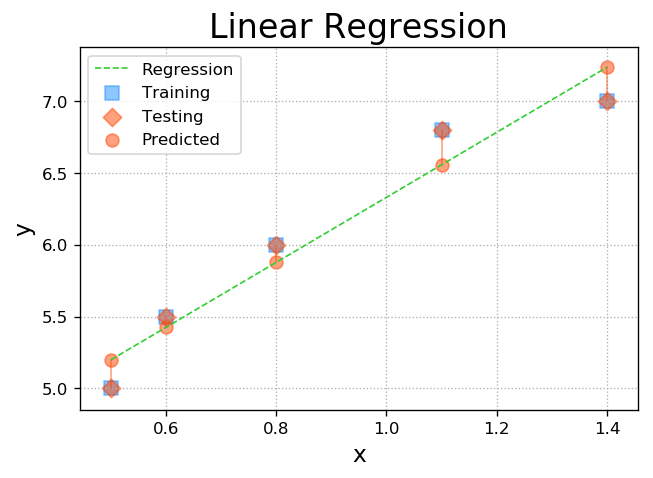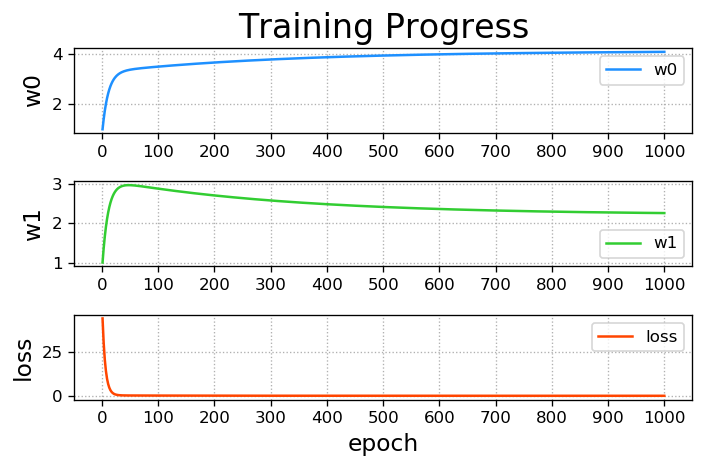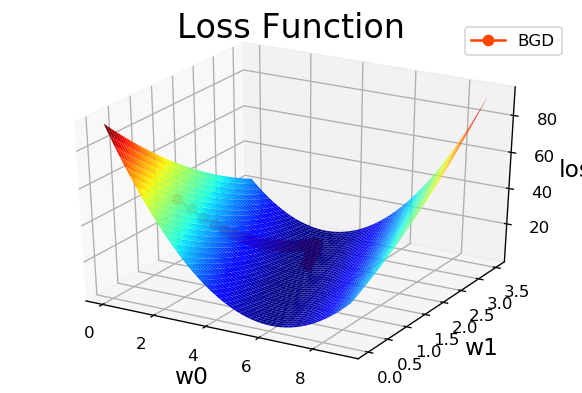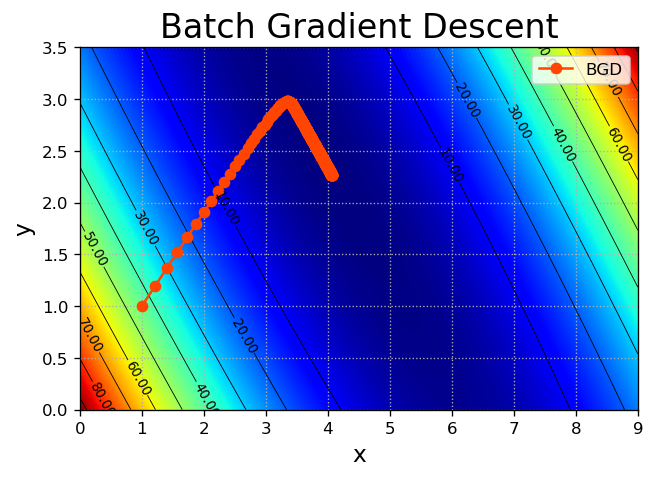6. 工具包
import sklearn.linear_model as lm
线性回归器 = lm.LinearRegression()
线性回归器.fit(已知输入, 已知输出)     # 计算模型参数
线性回归器.predict(新的输入)          ->新的输出

import pickle  #  保存模型

import numpy as np
import sklearn.linear_model as lm
import sklearn.metrics as sm
import matplotlib.pyplot as mp
import pickle

# 读取数据流程
x, y = [], []
with open('../data/single.txt', 'r') as f:
data = [float(substr) for substr in line.split(',')]
x.append(data[:-1])
y.append(data[-1])

# 转换为array
x = np.array(x)
y = np.array(y)

# 载入模型
# with open('../data/linear.pkl', 'rb') as f:

# 建立线性模型
model = lm.LinearRegression()
# 计算模型参数
model.fit(x, y)
# 预测新的输出
pred_y = model.predict(x)
# 查看效果1/(1+E) 误差越大，越接近0；误差越小，接近1
print(sm.r2_score(y, pred_y))

# 保存模型
# with open('../../data/linear.pkl', 'wb') as f:
#     pickle.dump(model, f)

# 绘制回归图形
mp.figure('Linear Regression', dpi=120)
mp.title('Linear Regression', fontsize=20)
mp.xlabel('x', fontsize=14)
mp.ylabel('y', fontsize=14)
mp.tick_params(labelsize=10)
mp.grid(linestyle=':')
mp.scatter(x, y, c='dodgerblue', alpha=0.75, s=60, label='Sample')
# 将x变为一维数组，按照排序绘制点
sorted_indices = x.ravel().argsort()
mp.plot(x[sorted_indices], pred_y[sorted_indices], c='orangered', label='Regression')

mp.legend()
mp.show()

0.7362638998481811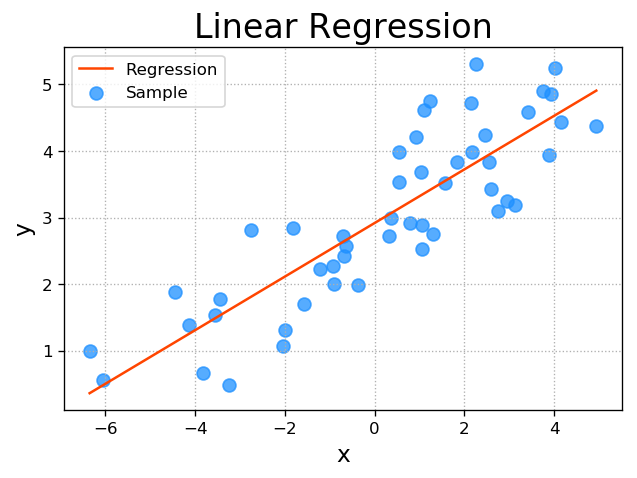五、岭回归
通过正则的方法，即在损失函数中加入正则项，以减弱模型参数对熟练数据的匹配度，借以规避少数明显偏移正常范围的异常样本影响模型的回归效果。

L

o

s

s

(

w

0

,

w

1

)

=

∑

(

y

−

(

w

0

+

w

1

x

)

)

2

2

+

正

则

强

度

×

f

(

w

0

,

w

1

)

Loss(w_0,w_1)=\sum{\frac{(y-(w_0+w_1x))^2}{2}}+正则强度 \times f(w_0,w_1)

lm.Ridge(300, fit_intercept=True) (正则强度，是否约束)
# rdg.py
import numpy as np
import sklearn.linear_model as lm
import matplotlib.pyplot as mp

x, y = [], []
with open('../data/abnormal.txt', 'r') as f:
data = [float(substr) for substr
in line.split(',')]
x.append(data[:-1])
y.append(data[-1])
x = np.array(x)
y = np.array(y)

# 普通线性回归
model1 = lm.LinearRegression()
model1.fit(x, y)
pred_y1 = model1.predict(x)

# 岭回归，若正则强度为0，则为普通线性回归
model2 = lm.Ridge(300, fit_intercept=True)
model2.fit(x, y)
pred_y2 = model2.predict(x)

mp.figure('Linear & Ridge Regression', dpi=120)
mp.title('Linear & Ridge Regression', fontsize=20)
mp.xlabel('x', fontsize=14)
mp.ylabel('y', fontsize=14)
mp.tick_params(labelsize=10)
mp.grid(linestyle=':')

mp.scatter(x, y, c='dodgerblue', alpha=0.75, s=60, label='Sample')
sorted_indices = x.ravel().argsort()
mp.plot(x[sorted_indices], pred_y1[sorted_indices], c='orangered', label='Linear')
mp.plot(x[sorted_indices], pred_y2[sorted_indices], c='limegreen', label='Ridge')

mp.legend()
mp.show()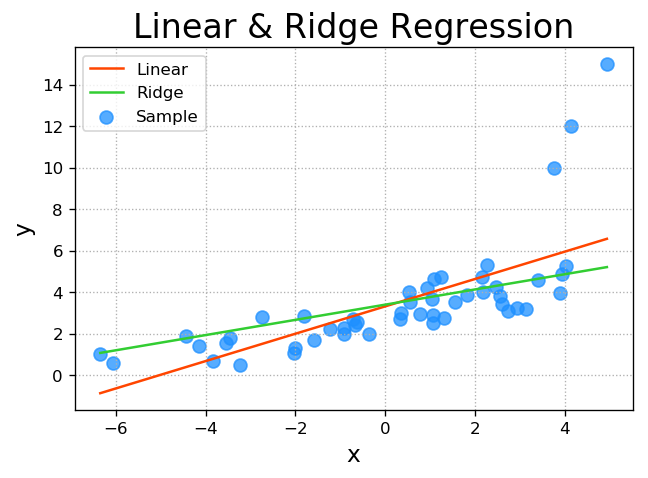六、多项式回归
多元线性：

y

=

w

0

+

w

1

x

1

+

w

2

x

2

+

w

3

x

3

+

.

.

.

+

w

n

x

n

y=w_0+w_1x_1+w_2x_2+w_3x_3+...+w_nx_n

将

x

2

,

x

3

.

.

.

x

n

x^2, x^3 ... x^n

看作

x

1

,

x

2

,

.

.

.

x_1, x_2, ...

一元多项式：

y

=

w

0

+

w

1

x

+

w

2

x

2

+

w

3

x

3

+

.

.

.

+

w

n

x

n

y=w_0+w_1x+w_2x^2+w_3x^3+...+w_nx^n

                                  x->多项式特征扩展器 -x1...xn-> 线性回归器->w0...wn
\______________________________________/
管线

# poly.py
import numpy as np
import sklearn.pipeline as pl
import sklearn.preprocessing as sp
import sklearn.linear_model as lm
import sklearn.metrics as sm
import matplotlib.pyplot as mp

train_x, train_y = [], []
with open('../data/single.txt', 'r') as f:
data = [float(substr) for substr
in line.split(',')]
train_x.append(data[:-1])
train_y.append(data[-1])

train_x = np.array(train_x)
train_y = np.array(train_y)
model = pl.make_pipeline(sp.PolynomialFeatures(10), lm.LinearRegression()) # 先sp预处理，参数为多项式最高次数，再使用lm方法
model.fit(train_x, train_y)
pred_train_y = model.predict(train_x)
print(sm.r2_score(train_y, pred_train_y))

test_x = np.linspace(train_x.min(), train_x.max(), 1000).reshape(-1, 1)
pred_test_y = model.predict(test_x)

mp.figure('Polynomial Regression', dpi=120)
mp.title('Polynomial Regression', fontsize=20)
mp.xlabel('x', fontsize=14)
mp.ylabel('y', fontsize=14)
mp.tick_params(labelsize=10)
mp.grid(linestyle=':')
mp.scatter(train_x, train_y, c='dodgerblue', alpha=0.75, s=60, label='Sample')
mp.plot(test_x, pred_test_y, c='orangered', label='Regression')

mp.legend()
mp.show()

0.7868629092058498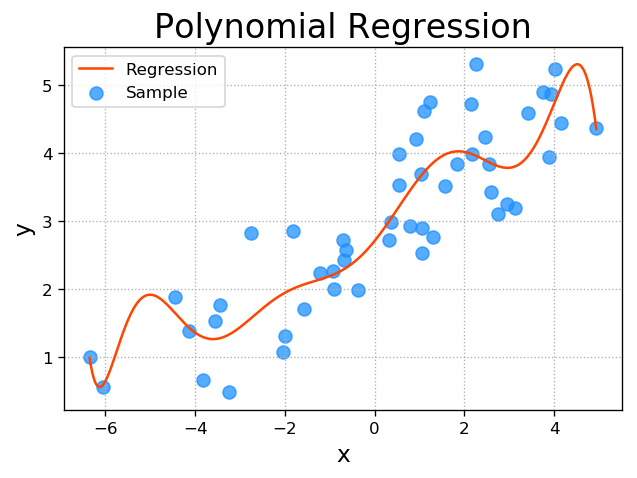展开全文一元线性回归
• 其实对于多元线性回归来说，x2,x3...x_2,x_3...x2​,x3​...是不同于x1=xx_1=xx1​=x的另一个特征，方程可表示为： y=θ1x1+θ2x2+...+θnxn+θ0y=\theta _{1}x_1+\theta _{2}x_2+...+\theta _{n}x_n+\theta _{0}y=...
1 概述
一元线性回归只能拟合

y

=

a

x

+

b

y=ax+b

，或者说只能拟合直线。 其实对于多元线性回归来说，

x

2

,

x

3

.

.

.

x_2,x_3...

是不同于

x

1

=

x

x_1=x

的另一个特征，方程可表示为：

y

=

θ

1

x

1

+

θ

2

x

2

+

.

.

.

+

θ

n

x

n

+

θ

0

y=\theta _{1}x_1+\theta _{2}x_2+...+\theta _{n}x_n+\theta _{0}

x

1

,

x

2

.

.

.

x_1,x_2...

是因变量（特征），

θ

1

,

θ

2

.

.

.

\theta _{1},\theta _{2}...

是系数，

θ

0

\theta _{0}

是截距。 多元线性回归可以用特征方程去求解。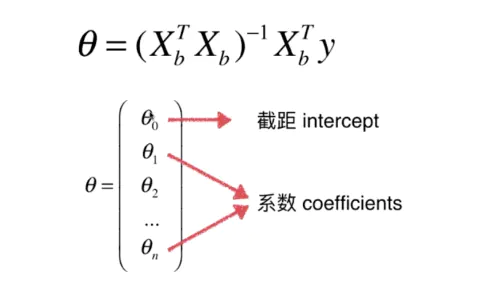代码实现：
import numpy as np

class LinearRegression():
def __init__(self):
self._theta = None
self.coef = None
self.interception = None

def fit(self, X_train, y_train):
X_b = np.hstack([np.ones((X_train.shape ,1)), X_train])

self._theta = np.linalg.inv(X_b.T.dot(X_b)).dot(X_b.T).dot(y_train)

self.coef = self._theta[1:]

self.interception = self._theta

return self

def predict(self, X_test):
X_b = np.hstack([np.ones((X_test.shape ,1)), X_test])
return X_b.dot(self._theta)

2 多项式线性回归
多项式线性回归是多元线性回归中比较特殊的一类，只有一个因变量

x

x

，其它的特征是

x

x

的几次方，比如

y

=

a

x

2

+

b

x

+

c

y=ax^2+bx+c

或者是更高次数的方程：

y

=

θ

1

x

+

θ

2

x

2

+

.

.

.

+

θ

n

x

n

+

θ

0

y=\theta _{1}x+\theta _{2}x^2+...+\theta _{n}x^n+\theta _{0}

以

y

=

a

x

2

+

b

x

+

c

y=ax^2+bx+c

为例：
import numpy as np
from matplotlib import pyplot as plt

x = np.random.uniform(-3, 3, size=100)
x = np.sort(x)
X = x.reshape(-1, 1)
# X.shape (100, 1)

y = 0.5*x**2 + x + 2 + np.random.normal(0, 1, 100)
# y.shape (100,)

plt.scatter(X, y)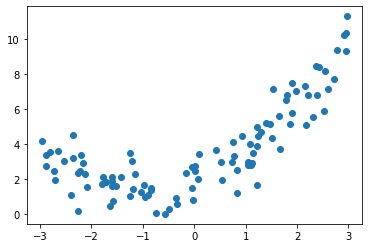这是一个抛物线，如果再用一元线性回归去拟合，就是下面的样子，可以发现误差时非常大的：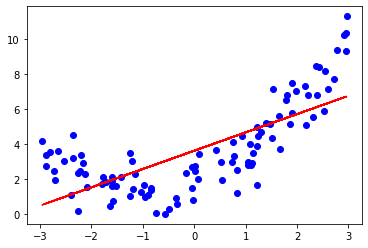一元线性回归只有一个特征

x

x

，多项式回归则是添加新的特征（

x

2

,

x

3

.

.

.

x^2, x^3...

），这里我只需要添加

x

x

的平方，来拟合一个抛物线。
3.1 使用np.hstack函数
# 使用np.hstack函数，将两个特征在水平方向上拼接
X2 = np.hstack([X, X**2]) # 拼接
# X2.shape (100, 2)

'''
注:  np.vstack() :在竖直方向上堆叠
np.hstack() :在水平方向上拼接
'''

from sklearn.linear_model import LinearRegression
lin_reg = LinearRegression()
lin_reg.fit(X2, y)
y_predict = lin_reg.predict(X2)
print(lin_reg.coef_) # 系数 a, b
# array([1.04078131, 0.54484198])
print(lin_reg.intercept_) # 截距 c
# 1.9212545662064286

# 绘制一下图像
plt.scatter(X, y, color="b")
plt.plot(x, y_predict, color="r")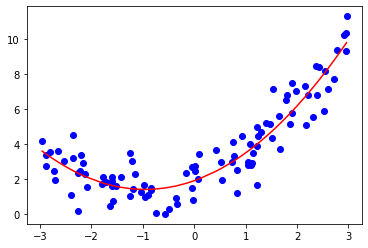3.2 PolynomialFeatures
from sklearn.preprocessing import PolynomialFeatures

# 构造多项式，如果只有一个x，degree=2，则为[1, x, x^2]，其中1代表截距列
poly = PolynomialFeatures(degree=2)

poly.fit(X)

X3 = poly.transform(X) # 构建X
X3.shape  # （100，3）


同样的，用LinearRegression()拟合。
lin_reg = LinearRegression()
lin_reg.fit(X3, y)
y_predict = lin_reg.predict(X3)

3.3 pipeline

from sklearn.pipeline import  Pipeline
from sklearn.preprocessing import StandardScaler

# 三合一
poly_reg = Pipeline([
("ploy", PolynomialFeatures(degree=2)), # 生成多项式特征
("std_scaler", StandardScaler()),       # 数据归一化
("lin_reg", LinearRegression())         # 进行线性回归
])

poly_reg.fit(X, y)
y_predict = poly_reg.predict(X)

plt.scatter(X, y, color="b")
plt.plot(x, y_predict, color="r")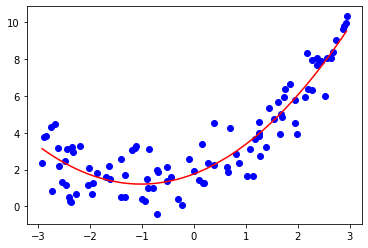展开全文• 机器学习2：多元线性回归~多项式回归 ~标准方程法 ~特征缩放与交叉验证 ~过拟合正则化 多元线性回归 原理 算法实现 手工实现 import numpy as np import matplotlib.pyplot as plt from mpl_...机器学习 标准方程法 特征缩放
• 文章内容：使用三次多项式对y = sin2πx进行插值。下面只涉及解题思路，不涉及相关概念。 一、解题思路 二、数据处理 三、运行结果 1.三次多项式系数 2.插值结果与y = sin2πx的比较 四、代码实现...机器学习 matlab
• 在一元线性回归中，输入特征只有一维， hθ=θ0+x1θ1h_{θ}=θ_0 + x_1θ_1, 对于多元特征，假设...对于非线性的一维数据，用线性回归拟合结果并不好，可以采用多项式回归，手动增加特征，例如如下4种多项式拟合 hθ
• 多元线性回归：  通过尺寸预测房价，只有尺寸一个特征，如果有多个特征比如楼层、房间数等，则 1. Hypothesis：假设（输入到输出的映射） 2. Parameters：参数/特征权重 3. cost function：代价函数 4....
• 1. 简单线性回归 相关系数： 相关系数| ρXY |=0时，则称X,Y不相关; | ρXY |=1时，则称X,Y完全相关，此时，X,Y之间具有线性函数关系; 当| ρXY | < 1时，X的变动引起Y的部分变动，ρXY的绝对值越大，X的变动...
• 一个简单线性回归和多项式回归在R中的实现示例常见线性回归的原理就不多说了，大家都懂，就是普通最小二乘法(Ordinary Least Square，OLS)回归法，主要用于简单线性回归、多项式回归和多元线性回归。R语言中，拟合...
• 主要介绍了scikit-learn线性回归多元回归，多项式回归的实现，文中通过示例代码介绍的非常详细，对大家的学习或者工作具有一定的参考学习价值，需要的朋友们下面随着小编来一起学习学习吧...